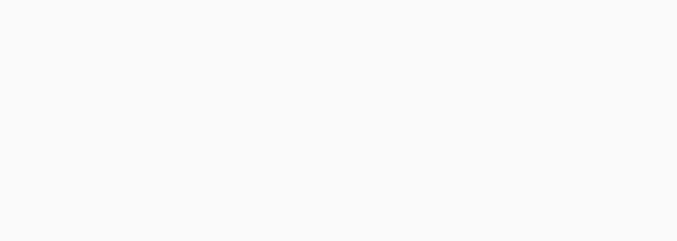# Rbse solution for Class 5 Maths Chapter 2 Shapes And Angles Solutions

ncert solution for Class 5 Maths Chapter 2 Shapes And Angles Solutions

Page: 16

Now you give the

(i) Is it a closed shape?

Solution:-

Yes, it is a closed shape.

(ii) Does it have 6 sides?

Solution:-

Yes, it has 6 sides.

But it is not the same as the one made by Rohini.

2. Mohini tried again.

(i) Is it a closed shape with 6 sides?

Solution:-

Yes, it is a closed shape with 6 sides.

(ii) Is it the same as the one made by Rohini?

Solution:-

No, it is not the same as the one made by Rohini.

(iii) Is there some way to say in what way these shapes are different?

Solution:-

These shapes are different in angles.

4. Look at the angles marked in these shapes. Can you see the difference?

Solution:-

By seeing the figure, we can say that the each figure has different shape and different angles.

Page: 18

1) Look at the shape and answer.

(i) The angle marked in colour is the biggest angle.

Solution:-

The angle marked in black colour is the biggest angle.

2.(a) Are the angles marked with yellow equal?

Solution:-

Yes, the angles marked with yellow are equal.

(b) Are the angles marked with green equal?

Solution:-

Yes, the angles marked with green are equal.

(c) Are the angles marked with blue equal?

Solution:-

Yes, the angles marked with blue are equal.

3. Four different angles are marked in four colours. Can you find other angles which are the same as the one marked in red? Mark them in red do this for other colours.

Solution:-

4. Look at the angles in the pictures and fill the table.

Solution:-

5. Sukhman made this picture with so many angles.

Use colour pencils to mark.

(i) right angles with black colour.

(ii) angles which are more than a right angle with green.

(iii) angles which are less than a right angle with blue.

Solution:-

6. Draw anything of your choice around the angle shown. Also write what kind of angle it is. The first one is done.

Solution:-

7. Write 3 names using straight lines and count the angles.

ncert solution for Class 5 Maths Chapter 2 Shapes And Angles Solutions

Solution:-

8.

These are two slides in a park.

(i) Which slide has a larger angle?

Solution:-

The slide which comes in left of the boy has larger angle.

(ii) Which slide do you think is safer for the little boy? Why?

Solution:-

The slide which comes in right is safer. Because, slide on the left of the boy is steeper than the other.

9. (i) Find out how many angles are there in each of these shapes. Mark them.

Solution:-

(ii) Find out and write your results in the table given.

Solution:-

10. There are many times in a day when the hands of a clock make a right angle. Now you draw some more.

Solution:-

11. Write what kind of angle is made by the hands at these times. Also write the time.

Solution:-

12. Draw the hands of the clock when they make an angle which is less than a right angle. Also write the time.

Solution:-

13. Can you guess how many degrees is the angle which is?

(i) ½ of a right angle .

Solution:-

We know that, the right angle is equal to 90o.

Then, ½ of a right angle = ½ × 90o

= 1 × 45

= 45o

(ii) 1/3 of a right angle .

Solution:-

We know that, the right angle is equal to 90o.

Then, 1/3 of a right angle = 1/3 × 90o

= 1 × 30

= 30o

(iii) 2 times of a right angle.

Solution:-

We know that, the right angle is equal to 90o.

Then, 2 times of a right angle = 2 × 90o

= 180o

Patio umbrellas sold at Costco recalled after reports of fires गर्मी में लू लगने से बचाव करेंगे यह खाद्य पदार्थ, आज ही खाना करें शुरू ट्रेन के बीच में ही AC कोच क्यों लगाए जाते हैं? Rbse books for class 1 to 12 hindi medium 2021-22